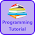# Programming Videos

## Search

### Find Second Largest Number in Array - C, C++ Program

Write a C,C++ program to find second largest element in an array.
All the element of an array is unique. So there is no repetition.

To find second highest element in  array whose element is repeated, check for the program to find the second highest element in an array with repeating element.

Logic of Program

1. Take input from user.

2. Sort the array using sorting algorithm such as bubble sort , QuickSort etc. I recommend you use algorithm which takes minimum time complexity for sorting such as QuickSort, MergeSort etc.

3. Once the array is sorted the element present in n-2 position is second largest element.

## Program to Find Second Largest Element in an Array

```#include <stdio.h>

void main()
{

int a,i,j,n,temp;

printf ("Enter the number of elements");
scanf ("%d",&n);

printf("Enter the values");

/* Taking input*/

for (i=0;i<n;i++){
scanf("%d",&a[i]);
}

/*Logic of sorting using bubble sort */

for(i=0;i<n;i++)
{
for(j=i+1;j<n;j++)
{
if(a[i]>a[j])
{
temp = a[i];
a[i]=a[j];
a[j]=temp;
}
}

}

// Now the array is sorted we know the position of second largest element

printf("Second largest element is %d",a[n-2]);

}

```

Find Second Highest Element in Single Traversal .

1.If the repeated highest value is given in the input then this code won't work. For e.g n=5 and the elemets given are 1,4,2,5,5. It will show the largest number is 5. :(

1.This program is for non-repeated array element. For repeated array element check this program http://programmingworldc.blogspot.in/2014/04/write-program-to-find-second-highest.html

2.Guys suppose the input was 3 4 2 4 5 1 5...then how can find out the second largest number in given array? Anyone can explain me

3.Hi, You can check this program to find second highest element in array (array which contains repeated element).

Find second highest element in an array .